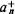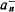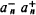# Commutation Relations

The following article is from The Great Soviet Encyclopedia (1979). It might be outdated or ideologically biased.

## Commutation Relations

fundamental relations in quantum mechanics that establish the connection between successive operations on the wave function, or state vector, of two operators (1 and L̂2) in opposite orders, that is, between 12 and 21. The commutation relations define the algebra of the operators. If the two operators commute, that is, 12 = L̂21, then the corresponding physical quantities L1 and L2 can have simultaneously defined values. But if their commutator is nonzero, that is, L̂L̂12 – L̂21 = c, then the uncertainty principle holds between the corresponding physical quantities: ΔL̂1ΔL̂2 ≤ ǀcǀ/2, where ΔL1 and ΔL2 are the uncertainties, or dispersions, of the measured values of the physical quantities L1 and L2. The commutation relation between the operator of the generalized coordinate and its conjugate generalized momentum (q̂p̂p̂ĝ = , where ħ is Planck’s constant) is very important in quantum mechanics. If the operator commutes with the operator of the total energy of the system (the Hamiltonian) , that is, L̂Ĥ = ĤL̂, then the physical quantity L (its average value, dispersion, and so on) preserves its value in time.

The commutation relations for the operators of the creation a+ and annihilation a of particles are of fundamental importance in the quantum mechanics of systems of identical particles and in quantum field theory. For a system of free (noninteracting) bosons, the particle creation operator in the state n,and the annihilation operator for the same particle,, satisfy the commutation relationfor fermions, the relationholds. The latter commutation relation is a formal expression of the Pauli principle.

V. B. BERESTETSKII

References in periodicals archive ?
 also used modified de Broglie relation approach to get the same commutation relations. When incorporating the GUP into quantum field theory, one needs to generalize D-dimensional deformed commutation relations to include time.
And, as we have known, the study of commutation relations between position and momentum operators is explained using Heisenberg uncertainty principle , which is given by
Commutation Relations, Normal Ordering, and Stirling Numbers
The commutation relations for each of the above symmetry generators are listed in Table 1.
They cover the structure of the space of the physical states, inequivalent representations of the canonical commutation relations, the spontaneous breakdown of symmetry and the Goldstone theorem, the dynamical rearrangement of symmetry and macroscopic manifestations of quantum field theory, thermal field theory and trajectories in the space of the representations, selected topics in thermal field theory, topological defects as non-homogeneous condensates, and dissipation and quantization.
In this respect, the vector-operators [MATHEMATICAL EXPRESSION NOT REPRODUCIBLE IN ASCII] satisfy the Bose commutation relations as:
Indeed, making use of the commutation relations (2), we can express any variable [a.sub.n], n [greater than or equal to] 2, in terms of [??], [a.sub.1] as the polynomial
Lounesto and Latvamaa  extended the Clifford algebra [Cl.sub.p,q] to the larger Clifford algebra [Cl.sub.p+1,q] and found simple commutation relations in [Cl.sub.=+1,q] describing the conformal Lie algebra of the conformal group on [R.sup.p,q].
The articles include Karasev's "Noncommutative Algebras, Nanostructures, and Quantum Dynamics Generated by Resonances," Karasev and Novikova's "Algebras with Polynomial Commutation Relations for a Quantum Particle in Electric and Magnetic Fields," Vorobjjev's "Poisson Structures and Linear Euler Systems over Symplectic Manifolds," and also his "Poisson Equivalence over a Symplectic Leaf."
Roy and Singh  and Li [11, 12] showed that the Pauli criterion cannot be applied in the time-dependent case: we note here that the inapplicability of the Pauli criterion means that (i) the KAM does not satisfy the fundamental commutation relations of the angular momentum and (ii) it reflects the breakdown of the symmetry of the electron's motion around the solenoid.
Equation (1) describes a particle with definite mass on condition that [[rho].sup.mu]] obey the Tzou commutation relations [20, 21, 23-25]:
The first is the canonical commutation relations of the infinite-dimensional Heisenberg algebra, or oscillator algebra.

Site: Follow: Share:
Open / Close